# July 2016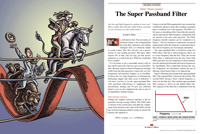For this month’s Traders’ Tips, the focus is John Ehlers’ article in this issue, “The Super Passband Filter.” Here, we present the July 2016 Traders’ Tips code with possible implementations in various software.

The Traders’ Tips section is provided to help the reader implement a selected technique from an article in this issue or another recent issue. The entries here are contributed by software developers or programmers for software that is capable of customization.In “The Super Passband Filter” in this issue, author John Ehlers describes a new oscillator he’s developed to help minimize computational lag. Ehlers calls this new oscillator a super passband filter. He has designed it to reject very low-frequency components and thus display as an oscillator as well as reject high-frequency components so as to minimize noise.

For convenience, we are providing TradeStation code for super passband filter indicators. In addition, we are providing EasyLanguage code for a TradeStation strategy based on the super passband filter calculation.

```//Super Passband Filter
// (c) 2016 John F. Ehlers
// TASC JUL 2016
inputs:
Period1( 40 ),
Period2( 60 ) ;
variables:
a1( 0 ),
a2( 0 ),
PB( 0 ),
count( 0 ),
RMS( 0 ) ;

a1 = 5 / Period1 ;
a2 = 5 / Period2 ;

PB = (a1 - a2) * Close + (a2*(1 - a1) - a1 * (1 - a2))
* Close + ((1 - a1) + (1 - a2))*PB - (1 - a1)
* (1 - a2)*PB ;

RMS = 0;
for count = 0 to 49
begin
RMS = RMS + PB[count]*PB[count] ;
end ;

RMS = SquareRoot( RMS / 50 ) ;

Plot1( PB, "Super PB" ) ;
Plot2( 0, "Zero Line" ) ;
Plot3( RMS, "+RMB" ) ;
Plot7(-RMS, "-RMS" ) ;

Strategy: Super Passband Filter

//Super Passband Filter
// (c) 2016 John F. Ehlers
// TASC JUL 2016
inputs:
Period1( 40 ),
Period2( 60 ),
UseZeroLineTarget( true ),
UseReversalStop( true );

variables:
a1( 0 ),
a2( 0 ),
PB( 0 ),
count( 0 ),
RMS( 0 ) ;

a1 = 5 / Period1 ;
a2 = 5 / Period2 ;

PB = (a1 - a2) * Close + (a2*(1 - a1) - a1 * (1 - a2))
* Close + ((1 - a1) + (1 - a2))*PB - (1 - a1)
* (1 - a2)*PB ;

RMS = 0;
for count = 0 to 49
begin
RMS = RMS + PB[count]*PB[count] ;
end ;

RMS = SquareRoot( RMS / 50 ) ;

if PB crosses over -RMS then
else if PB crosses under RMS then
Sell Short next bar at Market ;

if UseZeroLineTarget then
begin
if PB crosses over 0 then
Sell next bar at Market
else if PB crosses under 0 then
Buy to cover next bar at Market ;
end ;

If UseReversalStop then
begin
if PB crosses under -RMS then
Sell next bar at Market
else if PB crosses over RMS then
Buy to cover next bar at Market ;
end ;
```

A sample chart is shown in Figure 1.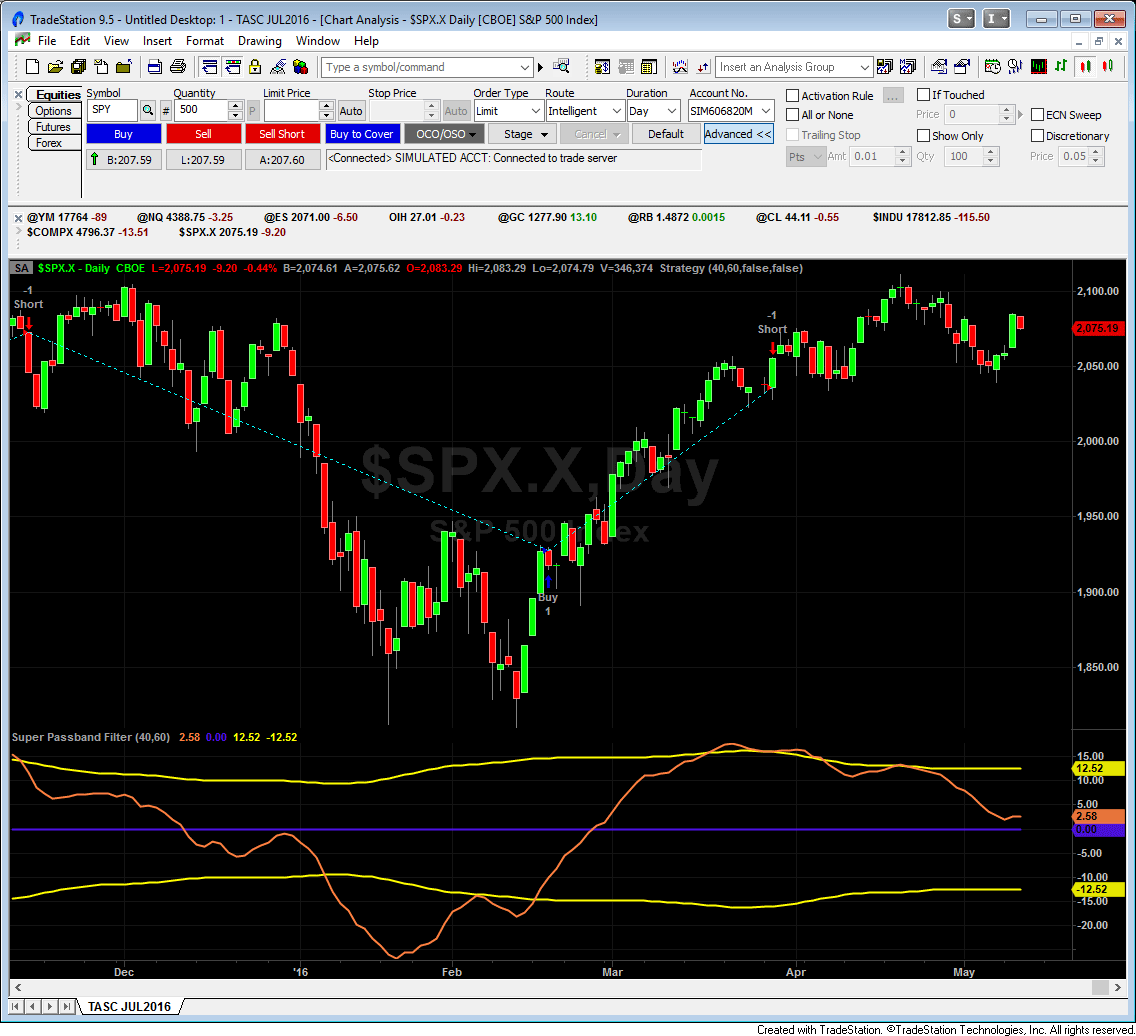FIGURE 1: TRADESTATION. Here is an example of the super passband filter indicator and strategy applied to a daily chart of the S&P 500 index.

—Doug McCrary

BACK TO LIST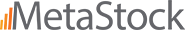### METASTOCK: JULY 2016

In “The Super Passband Filter” in this issue, John Ehlers presents his indicator called the super passband filter and suggests ways to trade it. The MetaStock formula given here for the indicator allows you to set whatever time periods are desired for the filter. The buy & sell signal formula has set those time periods as variables on the first two lines. If you wish to use different time periods, you must change those two lines in all four formulas or else the signals will not align.

#### Indicator:

```Passband Filter
p1:= Input("short time periods", 1, 100, 40);
p2:= Input("long time periods", 2, 200, 60);
a1:= 5/p1;
a2:= 5/p2;
PB:= (a1-a2)*C + (a2*(1-a1)-a1*(1-a2))*Ref(C,-1) +
((1-a1) + (1-a2))*PREV - (1-a1)*(1-a2)*Ref(PREV,-1);
RMSa:= Sum( pb*pb, 50);
RMS:= Sqrt(RMSa/50);
pb;
0;
RMS;
-RMS
```

```Enter Long:
p1:= 40;
p2:= 60;
a1:= 5/p1;
a2:= 5/p2;
PB:= (a1-a2)*C + (a2*(1-a1)-a1*(1-a2))*Ref(C,-1) +
((1-a1) + (1-a2))*PREV - (1-a1)*(1-a2)*Ref(PREV,-1);
RMSa:= Sum( pb*pb, 50);
RMS:= Sqrt(RMSa/50);
el:= Cross(pb,-RMS);
xl:= pb < Ref(pb, -1);
trade:= If(el, 1, If(xl, 0, PREV));

Exit Long:
p1:= 40;
p2:= 60;
a1:= 5/p1;
a2:= 5/p2;
PB:= (a1-a2)*C + (a2*(1-a1)-a1*(1-a2))*Ref(C,-1) +
((1-a1) + (1-a2))*PREV - (1-a1)*(1-a2)*Ref(PREV,-1);
RMSa:= Sum( pb*pb, 50);
RMS:= Sqrt(RMSa/50);
el:= Cross(pb,-RMS);
xl:= pb < Ref(pb, -1);
trade:= If(el, 1, If(xl, 0, PREV));

Enter Short:
p1:= 40;
p2:= 60;
a1:= 5/p1;
a2:= 5/p2;
PB:= (a1-a2)*C + (a2*(1-a1)-a1*(1-a2))*Ref(C,-1) +
((1-a1) + (1-a2))*PREV - (1-a1)*(1-a2)*Ref(PREV,-1);
RMSa:= Sum( pb*pb, 50);
RMS:= Sqrt(RMSa/50);
es:= Cross(RMS,pb);
xs:= pb > Ref(pb, -1);
trade:= If(es, 1, If(xs, 0, PREV));

Exit Short:
p1:= 40;
p2:= 60;
a1:= 5/p1;
a2:= 5/p2;
PB:= (a1-a2)*C + (a2*(1-a1)-a1*(1-a2))*Ref(C,-1) +
((1-a1) + (1-a2))*PREV - (1-a1)*(1-a2)*Ref(PREV,-1);
RMSa:= Sum( pb*pb, 50);
RMS:= Sqrt(RMSa/50);
es:= Cross(RMS,pb);
xs:= pb > Ref(pb, -1);
trade:= If(es, 1, If(xs, 0, PREV));
```

—William Golson
MetaStock Technical Support
www.metastock.com

BACK TO LIST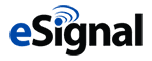### eSIGNAL: JULY 2016

For this month’s Traders’ Tip, we’ve provided the study SuperPassband.efs based on the formula described in John Ehlers’ article in this issue, “The Super Passband Filter.” In the article, Ehlers presents a method for filtering data and how to apply the study to your trading.

The study contains formula parameters that may be configured through the edit chart window (right-click on the chart and select “edit chart”). A sample chart is shown in Figure 2.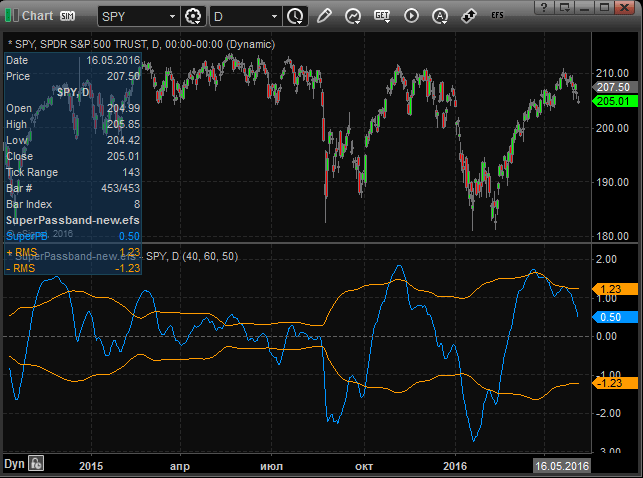FIGURE 2: eSIGNAL. Here is an example of the SuperPassband study plotted on a daily chart of SPY.

To discuss this study or download a complete copy of the formula code, please visit the EFS Library Discussion Board forum under the forums link from the support menu at www.esignal.com or visit our EFS KnowledgeBase at http://www.esignal.com/support/kb/efs/. The eSignal formula script (EFS) is also available for copying & pasting below.

```/*********************************
Provided By:
eSignal (Copyright c eSignal), a division of Interactive Data
Formula Script (EFS) is for educational purposes only and may be
modified and saved under a new file name.  eSignal is not responsible
for the functionality once modified.  eSignal reserves the right
to modify and overwrite this EFS file with each new release.

Description:
The Super Passband Filter by John F. Ehlers

Version:            1.00  05/05/2016

Formula Parameters:                     Default:
EMA Short Period                        40
EMA Long Period                         60
RMS Period                              50

Notes:
The related article is copyrighted material. If you are not a subscriber

**********************************/

var fpArray = new Array();

function preMain(){

setPriceStudy(false);
setCursorLabelName("SuperPB", 0);
setDefaultBarFgColor(Color.RGB(0,148,255), 0);
setCursorLabelName("+ RMS", 1);
setDefaultBarFgColor(Color.RGB(255,155,0), 1);
setCursorLabelName("- RMS", 2);
setDefaultBarFgColor(Color.RGB(255,155,0), 2);

var x=0;
fpArray[x] = new FunctionParameter("EMAShort", FunctionParameter.NUMBER);
with(fpArray[x++]){
setName("EMA Short Period");
setLowerLimit(1);
setDefault(40);
}
fpArray[x] = new FunctionParameter("EMALong", FunctionParameter.NUMBER);
with(fpArray[x++]){
setName("EMA Long Period");
setLowerLimit(1);
setDefault(60);
}
fpArray[x] = new FunctionParameter("RMSPeriod", FunctionParameter.NUMBER);
with(fpArray[x++]){
setName("RMS Period");
setLowerLimit(1);
setDefault(50);
}
}

var bInit = false;
var bVersion = null;
var a1 = 0;
var a2 = 0;
var xClose = null;
var xPB = null;
var xRMS = null;

function main(EMAShort, EMALong, RMSPeriod) {

if (bVersion == null) bVersion = verify();
if (bVersion == false) return;

if(getBarState() == BARSTATE_ALLBARS){
bInit = false;
}

if (getCurrentBarCount() < EMALong) return;

if (!bInit){
a1 = 5 / EMAShort;
a2 = 5 / EMALong;
xClose = close();
xPB = efsInternal("Calc_PassBand", xClose, a1, a2);
xRMS = efsInternal("Calc_RMS",xPB, RMSPeriod);
bInit = true;
}

if (xPB.getValue(0) == null || xRMS.getValue(0) == null) return;

return [xPB.getValue(0), xRMS.getValue(0), (-xRMS.getValue(0))];

}

function Calc_PassBand(Close, a1, a2){

var PB = null;
var PB_1 = ref(-1);
var PB_2 = ref(-2);

PB = (a1 - a2) * Close.getValue(0)
+ (a2 * (1-a1) - a1 * (1 - a2)) * Close.getValue(-1)
+ ((1 - a1) + (1 - a2)) * PB_1 - (1 - a1)*(1 - a2) * PB_2;

return PB;
}

function Calc_RMS(PB, Period){

var RMS = null;
for (var i = 0; i < Period; i++){
RMS = RMS + PB.getValue(-i) * PB.getValue(-i);
}
RMS = Math.sqrt(RMS / Period);

return RMS;
}

function verify(){

var b = false;
if (getBuildNumber() < 779){

drawTextAbsolute(5, 35, "This study requires version 10.6 or later.",
Color.white, Color.blue, Text.RELATIVETOBOTTOM|Text.RELATIVETOLEFT|Text.BOLD|Text.LEFT,
null, 13, "error");
Color.white, Color.blue, Text.RELATIVETOBOTTOM|Text.RELATIVETOLEFT|Text.BOLD|Text.LEFT,
return b;
}
else
b = true;

return b;
}
```

—Eric Lippert
eSignal, an Interactive Data company
800 779-6555, www.eSignal.com

BACK TO LIST### THINKORSWIM: JULY 2016

In “The Super Passband Filter” in this issue, John Ehlers addresses the problem of frequencies in indicators that are too low or too high. The common practice to refine frequency is to enable smart filters. However, good filters take lots of computational power. So Ehlers shows us how to use filters while keep computing power relatively low.

We have recreated Ehlers’ study using our proprietary scripting language, thinkscript. We have made the loading process extremely easy. Simply click on the link http://tos.mx/zeAlsQ and choose view thinkScript. Choose to rename your study EhlersSuperPassbandFilter. You can adjust the parameters of this strategy within the edit studies window to fine-tune your variables.

In Figure 3, you see a chart of SPY, the ETF built to track the S&P 500, along with the EhlersSuperPassbandFilter added. For use of the study, traders should focus on the red line crossing one of the yellow lines. For more information about the filter, please refer to Ehlers’ article in this issue.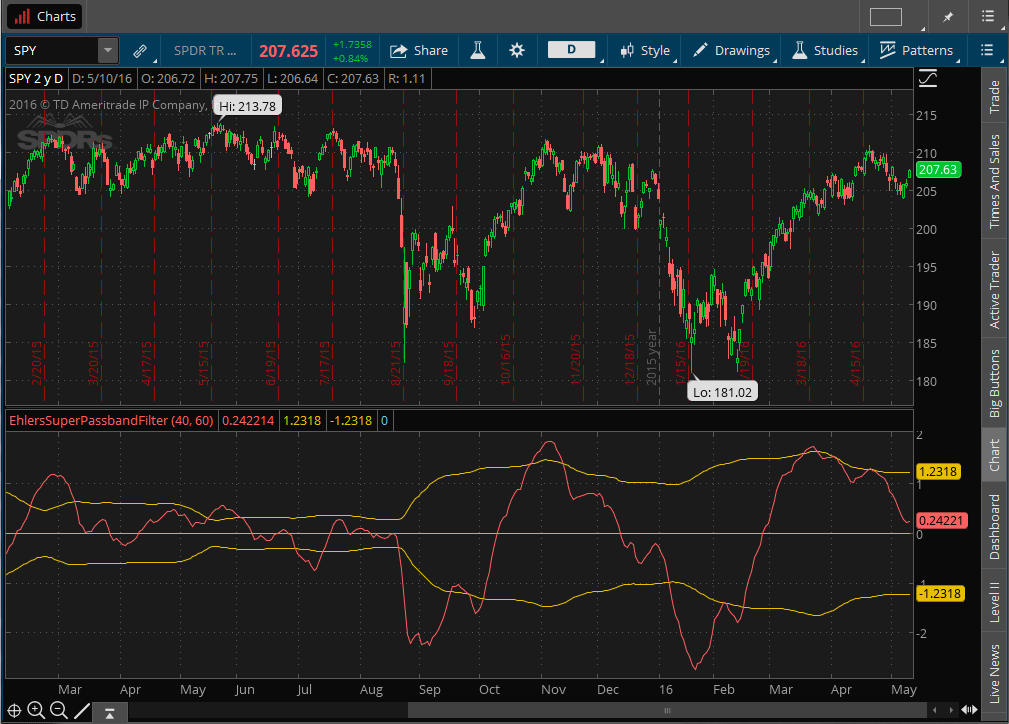FIGURE 3: THINKORSWIM. Here is a chart of SPY along with the EhlersSuperPassbandFilter added, showing the red line crossing one of the yellow lines.

—thinkorswim
A division of TD Ameritrade, Inc.
www.thinkorswim.com

BACK TO LIST### WEALTH-LAB: JULY 2016

Author John Ehlers continues to please readers with more nearly-zero lag filters—this time, it’s the super passband oscillator, which he describes in his article in this issue, “The Super Passband Filter.”

Following the trading idea in his article, we are presenting a simple WealthScript system that can be applied selectively (only long side or both sides):

• Buy on the filter crossing above its -RMS line
• Short on the filter crossing below its RMS line
• Exit long when the filter either crosses below its RMS or crosses below -RMS (which signifies a false entry signal)
• Cover short when the filter either crosses above its -RMS or crosses above RMS (which signifies a false entry signal)

Our tests suggest that this new nearly-zero lag filter looks promising for countertrend trades and for buying on significant dips (see Figure 4). To execute this trading system, Wealth-Lab users need to install (or update) the latest version of the TASCIndicators library from the extensions section of our website if they haven't already done so, and then restart Wealth-Lab.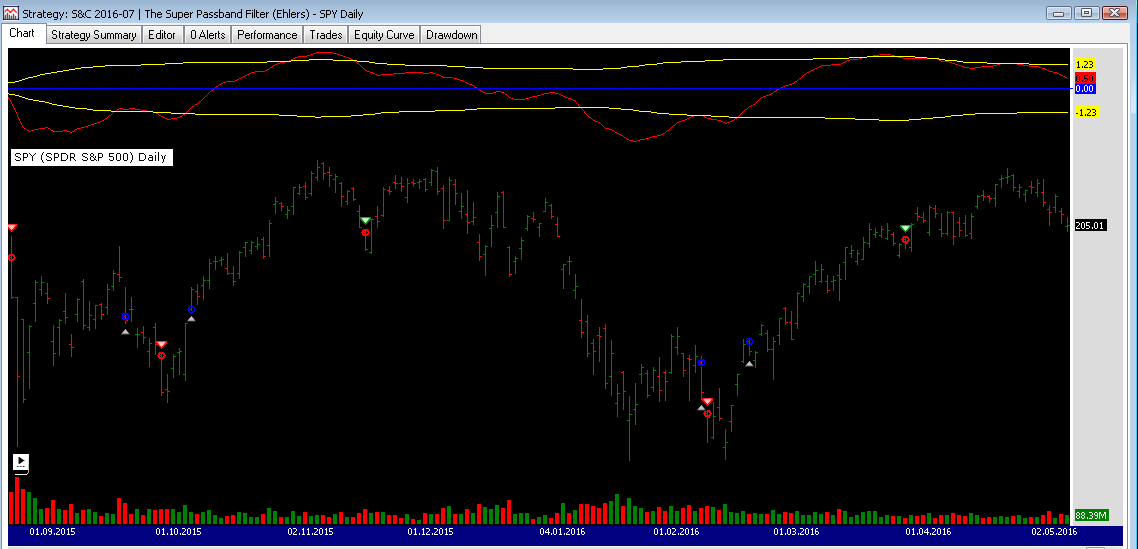Figure 4: WEALTH-LAB. This sample Wealth-Lab 6 chart illustrates entries made by a system based on John Ehlers’ super passband filter.

#### Wealth-Lab Code

```using System;
using System.Collections.Generic;
using System.Text;
using System.Drawing;
using WealthLab;
using WealthLab.Indicators;
using TASCIndicators;

namespace WealthLab.Strategies
{
public class TASC201607 : WealthScript
{
private StrategyParameter paramPeriod1;
private StrategyParameter paramPeriod2;
private StrategyParameter paramGoShort;

public TASC201607()
{
paramPeriod1 = CreateParameter("Period1", 40, 10, 100, 10);
paramPeriod2 = CreateParameter("Period2", 60, 20, 300, 10);
paramGoShort = CreateParameter("Go short?", 0, 0, 1, 1);
}

protected override void Execute()
{
int Period1 = paramPeriod1.ValueInt;
int Period2 = paramPeriod2.ValueInt;
bool goShort = paramGoShort.ValueInt == 1 ? true : false;

var spb = SuperPassband.Series(Close,40,60);
var rms = SuperPassbandRMS.Series(Close,40,60);
var mrms = rms*-1;

var pbPane = CreatePane(30,true,true);
PlotSeries(pbPane,spb,Color.Red,WealthLab.LineStyle.Solid,1);
DrawHorzLine(pbPane,0,Color.Blue,WealthLab.LineStyle.Solid,1);
PlotSeriesFillBand(pbPane,rms,mrms,Color.Yellow,Color.Transparent,WealthLab.LineStyle.Solid,1);

for(int bar = Math.Max(Period1,Period2); bar < Bars.Count; bar++)
{
SetBackgroundColor(bar,Color.Black);

if (IsLastPositionActive)
{
Position p = LastPosition;
if( p.PositionType == PositionType.Long )
{
if( CrossUnder(bar, spb, rms) || CrossUnder(bar, spb, mrms) )
SellAtMarket(bar+1, p);
}
else
{
if( CrossOver(bar, spb, mrms) || CrossOver(bar, spb, rms))
CoverAtMarket(bar+1, p);
}
}
else
{
if( CrossOver(bar, spb, mrms))
else
if( CrossUnder(bar, spb, rms) && goShort )
ShortAtMarket(bar+1);
}
}
}
}
}
```

—Eugene, Wealth-Lab team
MS123, LLC
www.wealth-lab.com

BACK TO LISTJohn Ehlers’ super passband oscillator described in his article in this issue, “The Super Passband Filter,” can be easily implemented with a few of NeuroShell Trader’s 800+ indicators. Simply select new indicator from the insert menu and use the indicator wizard to set up the following indicators:

```PB:	ExpAvg1-ExpAvg2(Close,16,24)
RMS:	SqrRt(Divide(Sum(Pow(PB,2),50),50))
-RMS:	Neg(RMS)
```

Users of NeuroShell Trader can go to the STOCKS & COMMODITIES section of the NeuroShell Trader free technical support website to download a copy of this or any previous Traders’ Tips.

A sample chart is shown in Figure 5.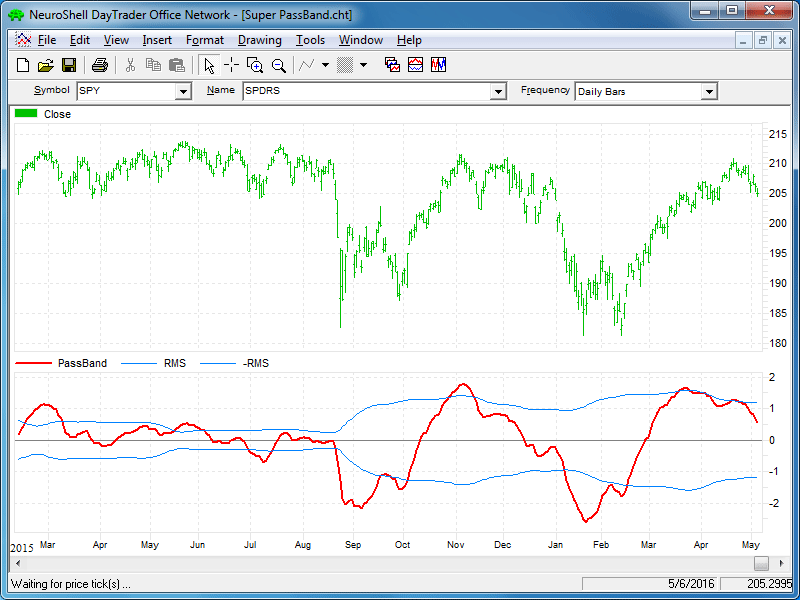FIGURE 5: NEUROSHELL TRADER. This sample NeuroShell Trader chart shows the PassBand, RMS and –RMS indicators.

—Marge Sherald, Ward Systems Group, Inc.
301 662-7950, sales@wardsystems.com
www.neuroshell.com

BACK TO LIST• Function: Ehlers_SPB—Returns the Ehlers Super PassBand values
• Function: Ehlers_RMS—Returns the Ehlers RMS value
• Indicator plot: Ehlers_SPB_Ind—Plots the Ehlers SPB indicator on a chart
• System: Ehlers_SPB_sys—An example, not in the article, created by Richard Denning, that shows how the indicator might be used in creating a trading system.

I tried various rules for the shorting but could not find one that returns a profit, consequently I added a parameter that shuts off the shorting. Figure 6 shows the equity curve for the example system (long only) from 1999 through 2014 trading one share per signal of the NASDAQ 100 stocks.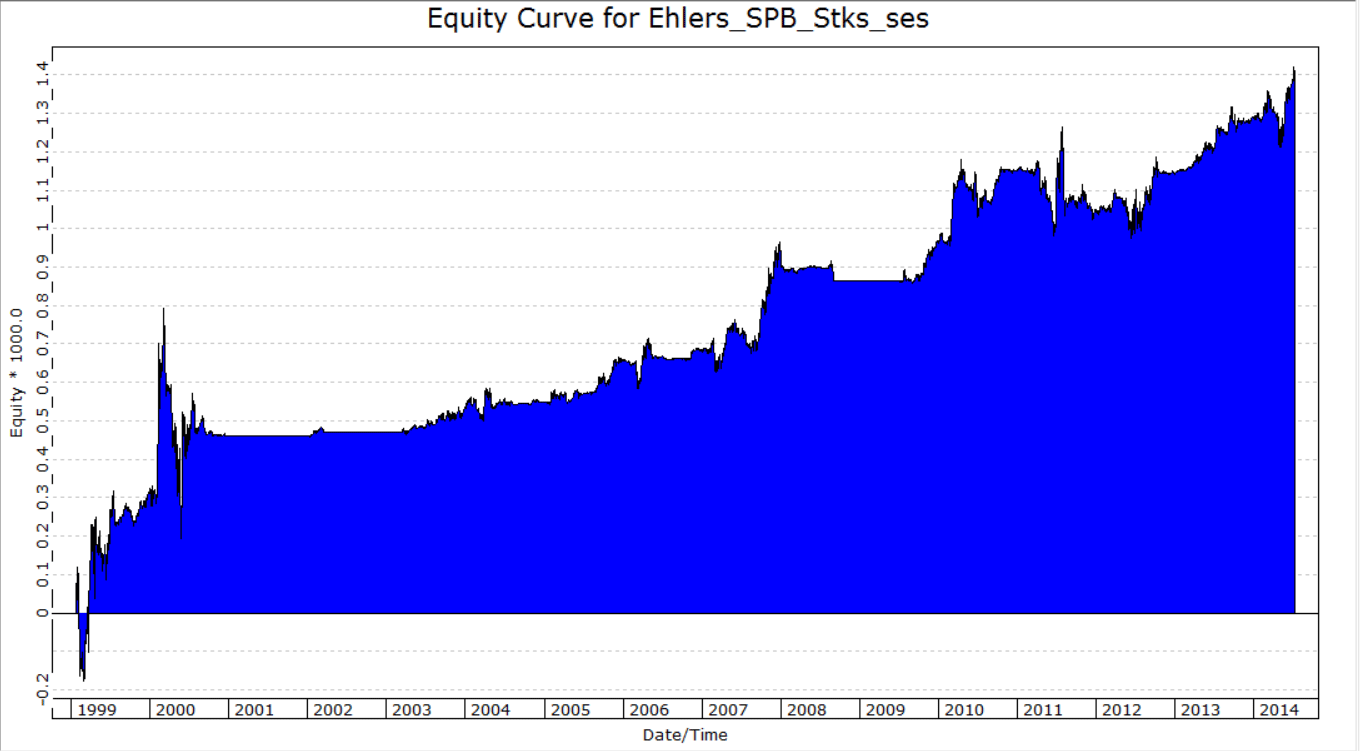FIGURE 6: TRADERSSTUDIO. Here is a sample equity curve for the system from 1999 through 2014 trading one share per signal of the NASDAQ 100 stocks.

```'THE SUPER PASBAND FILTER
'Author: John Ehlers, TASC July 2016
'Coded by: Richard Denning 5/11/16

'returns the Ehlers Super PassBand value:
Function Ehlers_SPB(Period1, Period2)
Dim a1
Dim a2
Dim PB As BarArray

a1 = 5 / Period1
a2 = 5 / Period2
PB = (a1 - a2)*Close + (a2*(1 - a1) - a1*(1 - a2))*Close + ((1 - a1) + (1 - a2))*PB - (1 - a1)*(1 - a2)*PB
Ehlers_SPB = PB
End Function
'-----------------------------------------------------------------------------------------------------------------------
'returns the Ehlers RMS value:
Function Ehlers_RMS(Period1,Period2)
Dim PB As BarArray
Dim RMS As BarArray
Dim count
PB = Ehlers_SPB(Period1,Period2)

RMS = 0
For count = 0 To 49
RMS = RMS + PB[count]*PB[count]
Next
RMS = Sqr(RMS / 50)
Ehlers_RMS = RMS
End Function
'-----------------------------------------------------------------------------------------------------------------------
'plots the Ehlers_SPB and RMS indicators:
Sub Ehlers_SPB_Ind(Period1,Period2)
plot1(Ehlers_SPB(Period1,Period2))
plot2(Ehlers_RMS(Period1,Period2))
plot3(-Ehlers_RMS(Period1,Period2))
plot4(0)
End Sub
'------------------------------------------------------------------------------------------------------------------------
'example system created by Richard Denning using the Ehlers SPB & RMS indicators:
'Note the following system is not in the article but is an example
'   of how the indicator might be used in a system
Sub Ehlers_SPB_sys(Period1,Period2,TrendLen,longOnly)
'default: Period1=40, Period2=60, longOnly=1
Dim myRMS As BarArray
Dim mySPB As BarArray
Dim NDX As BarArray
mySPB = Ehlers_SPB(Period1,Period2)
myRMS = Ehlers_RMS(Period1,Period2)
NDX = C Of independent1
If CrossesOver(mySPB,-myRMS)And C>Average(C,TrendLen) And NDX>Average(NDX,TrendLen) Then Buy("LE",1,0,Market,Day)
If CrossesUnder(mySPB,myRMS) Then ExitLong("LX","",1,0,Market,Day)
If CrossesUnder(mySPB,0) Then ExitLong("LX_SE","LE",1,0,Market,Day)
If CrossesUnder(mySPB,0) And longOnly<>1 And C<Average(C,TrendLen) And NDX<Average(NDX,TrendLen) Then Sell("SE",1,0,Market,Day)
If CrossesOver(mySPB,-myRMS) Then ExitShort("SX_myRMS","SE",1,0,Market,Day)
If CrossesOver(mySPB,0) Then ExitShort("SX_0","SE",1,0,Market,Day)
End Sub
'----------------------------------------------------------------------------------------------------------------------------------
```

—Richard Denning

BACK TO LIST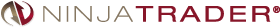The filter discussed in “The Super Passband Filter” by John Ehlers in this issue is available for download at www.ninjatrader.com/SC/July2016SC.zip.

You can review the indicators’ source code by selecting the menu Tools → Edit NinjaScript → Indicator from within the NinjaTrader Control Center window and selecting the SuperPassBandFile file.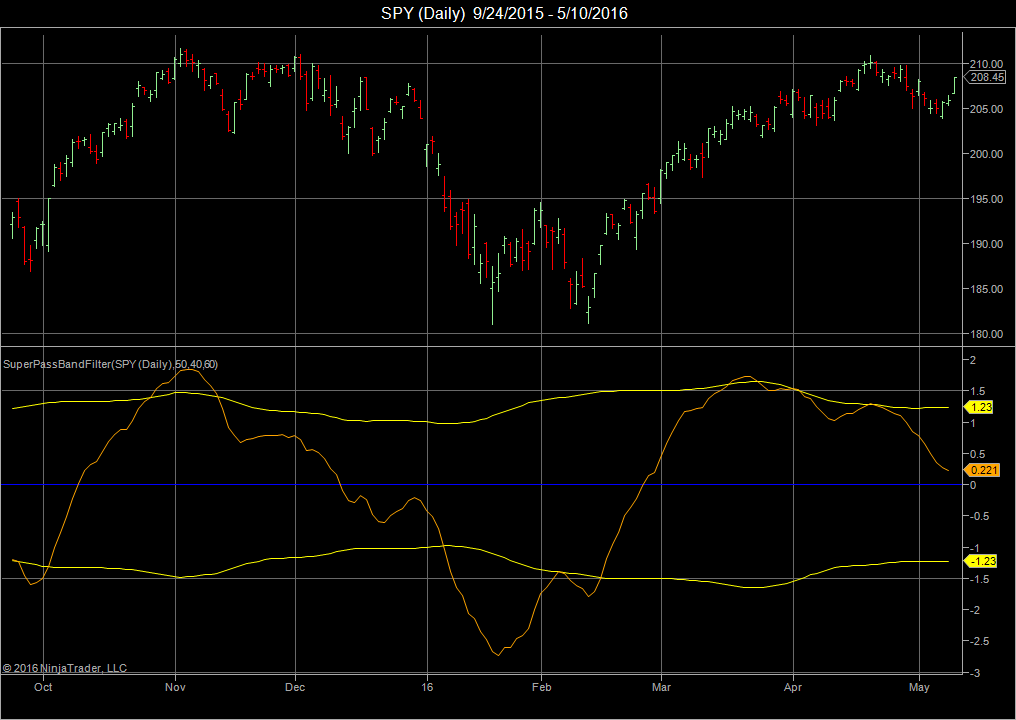FIGURE 7: NINJATRADER. The SuperPassBandFilter is displayed on an SPY daily chart ending in May 2016.

NinjaScript uses compiled DLLs that run native, not interpreted, which provides you with the highest performance possible.

—Raymond Deux & Jess Pennell

BACK TO LIST### UPDATA: JULY 2016

Our Traders’ Tip for this month is based on “The Super Passband Filter” in this issue by John Ehlers.

In the article, Ehlers seeks to filter out both high and low frequencies from market data, eliminating distracting “wiggles” from the resultant signal with minimal lag effect. This indicator achieves this with two differenced exponential moving averages (EMAs) of varying periods. Trigger points for the filter are added with a root mean square (RMS) envelope over the signal line.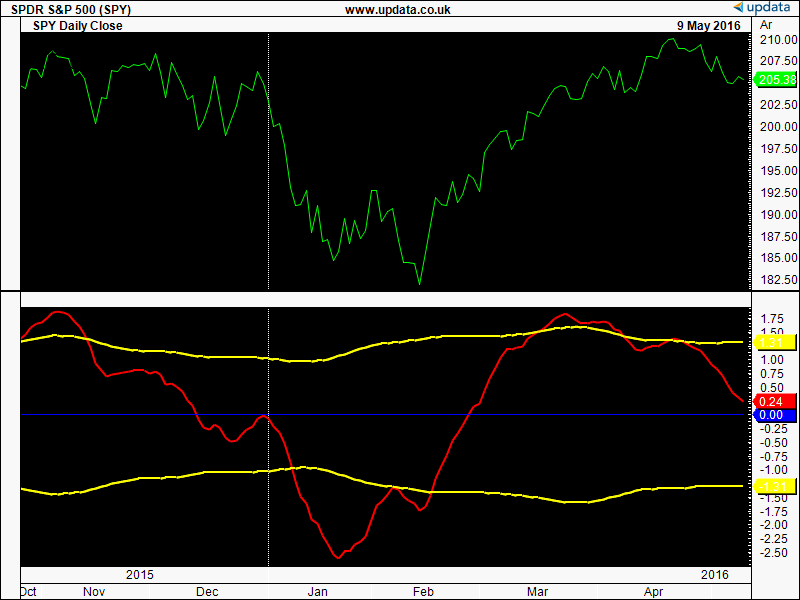FIGURE 8: UPDATA. Here, the super passband filter is applied to the SPY ETF of daily resolution.

The Updata code for this article can be found in the Updata library and may be downloaded by clicking the custom menu and indicator Library. Those who cannot access the library due to a firewall may paste the code shown here into the Updata custom editor and save it.

```'Super PassBand Filter
PARAMETER "Period 1" @PERIOD1=40
PARAMETER "Period 2" @PERIOD2=60
NAME "" ""
DISPLAYSTYLE 4LINES
INDICATORTYPE CHART
PLOTSTYLE THICK2 RGB(255,0,0)
PLOTSTYLE2 THICK2 RGB(255,255,0)
PLOTSTYLE3 THICK2 RGB(255,255,0)
COLOUR4 RGB(0,0,255)
@A1=0
@A2=0
@PB=0
#COUNT=0
@RMS=0

FOR #CURDATE=MAX(@PERIOD1,@PERIOD2) TO #LASTDATE
@A1=5/@PERIOD1
@A2=5/@PERIOD2
@PB=(@A1-@A2)*CLOSE+(@A2*(1-@A1)-@A1*(1-@A2))*CLOSE(1)+((1-@A1)+(1-@A2))*HIST(@PB,1)      ...-((1-@A1)*(1-@A2)*HIST(@PB,2))
@RMS=0
FOR #COUNT=0 TO 49
@RMS=@RMS+(HIST(@PB,#COUNT)*HIST(@PB,#COUNT))
NEXT
@PLOT=@PB
@PLOT2=EXPBASE(@RMS/50,0.5)
@PLOT3=-EXPBASE(@RMS/50,0.5)
@PLOT4=0
NEXT
```

—Updata support team
support@updata.co.uk
www.updata.co.uk

BACK TO LIST### AMIBROKER: JULY 2016

In “The Super Passband Filter” in this issue, John Ehlers presents a passband filtering technique based on subtraction of two exponential moving averages (EMAs). It is worth noting that mathematically, such a filter is similar to the classic MACD indicator. The difference is the way that the smoothing factor is calculated. Ehlers uses a smoothing factor of 5/period while the classic EMA uses factor of 2/(period+1). So the relationship between passband filter parameters and the classic MACD is given by:

`PeriodMACD = -1 + PeriodPassBand/2.5;`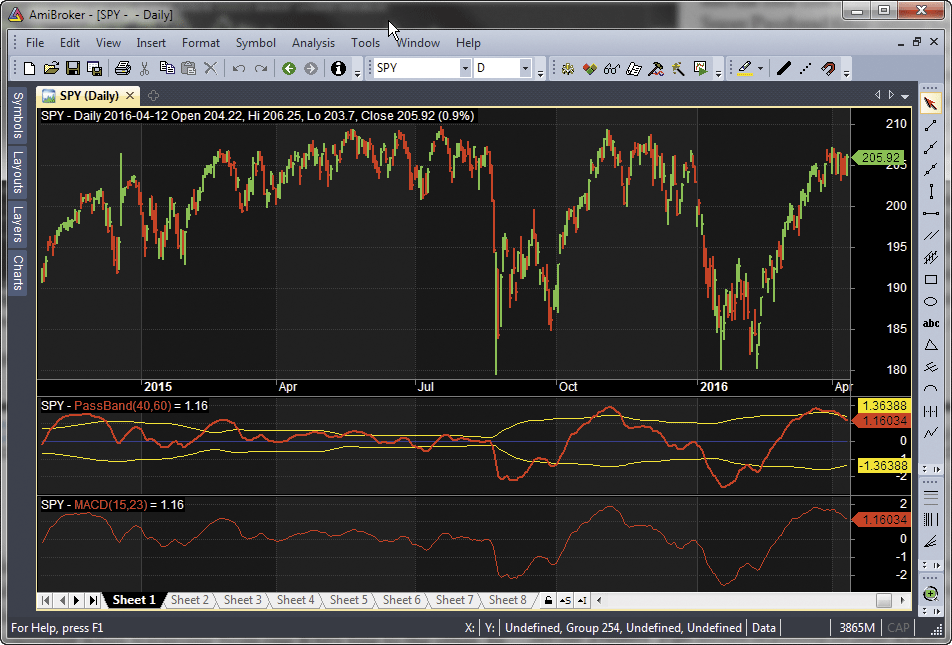FIGURE 9: AMIBROKER. The Ehlers super passband (red) is shown in the middle pane together with RMS lines (yellow). A classic MACD with periods of 15 and 23 is shown in the bottom pane. Both produce the same values.

A ready-to-use formula that implements the super passband filter as described in Ehlers’ article is shown here. To use the formula, enter the code in the formula editor and press apply to display the passband indicator.

```AmiBroker code
Period1 = Param("Period1", 40, 1, 100 );
Period2 = Param("Period2", 60, 1, 100 );

a1 = 5 / Period1;
a2 = 5 / Period2;

// pass band by difference of EMA's
// essentially it is 're-invented' MACD
PB = AMA( C, a1 ) - AMA( C, a2 );

RMS = sqrt( MA( PB * PB, 50 ) );

Plot( PB, "PassBand" + _PARAM_VALUES(), colorRed, styleThick );
Plot( RMS, "", colorYellow );
Plot( -RMS, "", colorYellow );
Plot( 0, "", colorBlue, styleNoLabel );
```

—Tomasz Janeczko, AmiBroker.com
www.amibroker.com

BACK TO LIST

### MICROSOFT EXCEL: JULY 2016

In “The Super Passband Filter” in this issue, author John Ehlers presents an indicator with a very quick reaction profile.

By replicating Figure 3 from Ehlers’ article (Figure 10) and playing with the cursor, we can see that the lowest and highest points on the indicator are never more than one bar behind the lowest or highest closes, marking a change in significant localized trends. Hard to get much closer than that, eh?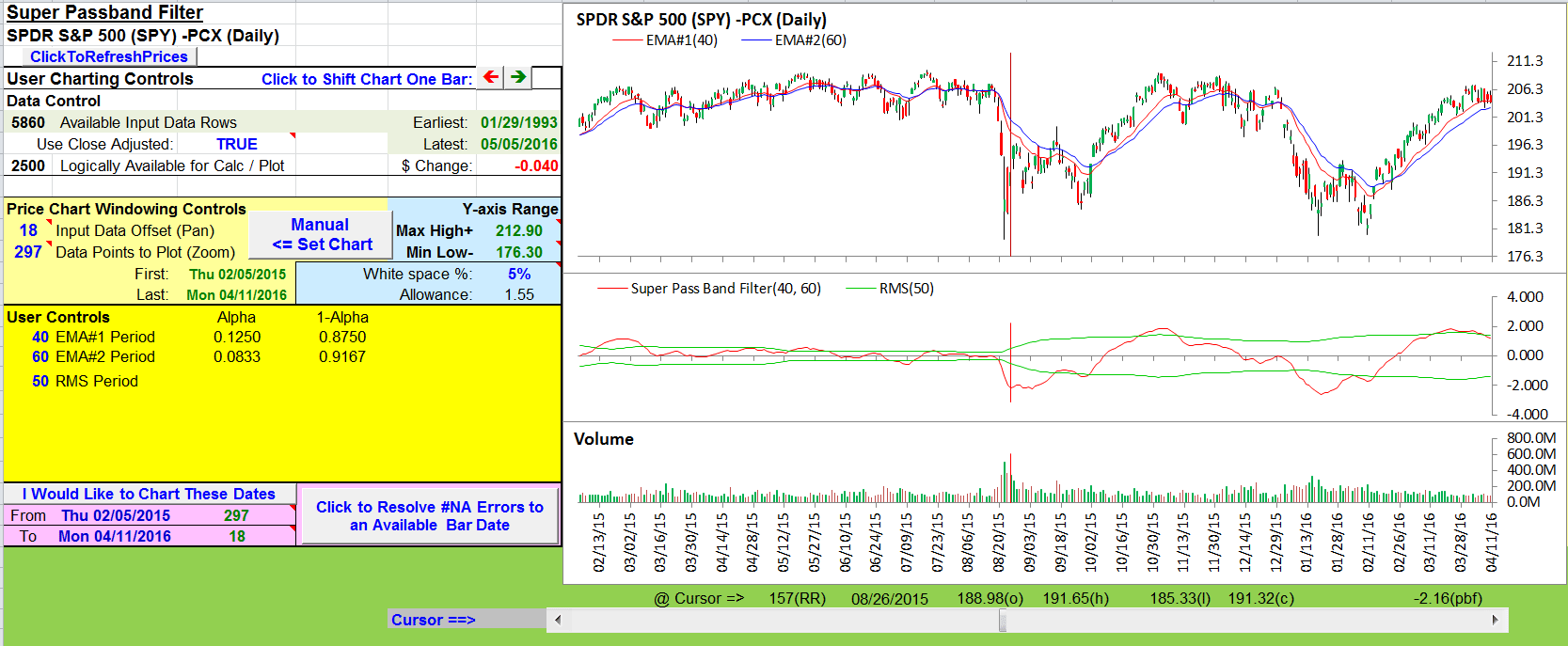FIGURE 10: EXCEL. Here is an approximation of Figure 3 from John Ehlers’ article in this issue, “The Super Passband Filter.”

Ehlers suggests using crossover points of the filter value and an RMS envelope of the super bandpass filter as a means of generating long or short entry signals.

For those crossovers where a trend appears to be in progress, this seems to work well. In sideways patterns where a trend is not well established, this technique can lead to whipsaws.

This indicator can give the trader a very early warning of trend changes. For safety, one should look for additional entry confirmations to control risk.

A note about the EMA calculations used in this article: In this filter, Ehlers is using a more aggressive alpha calculation to build his EMAs than is used in either of the perhaps more familiar EMA versions. This EMA is significantly more reactive to the most recent input value than a standard EMA or a Wilders’ EMA.

`Generalized EMA formula = price today * α + EMA yesterday * (1- α)`

Here is the formula for a more standard EMA, as found in many software packages:

```α = 2 / (N+1)
Using N = 40: α = 2 / (N+1) = 0.04878, and (1 - α) = 0.95121```

Here is the formula for Wilders’ EMA:

```α = 1/N
Using N = 40: α = 1/N = 0.02500, and (1 - α) = 0.97500```

And finally, here is the formuala as used in the super bandpass filter:

```α = 5/N
Using N = 40: α = 5/N = 0.12500, and (1 - α) = 0.87500```